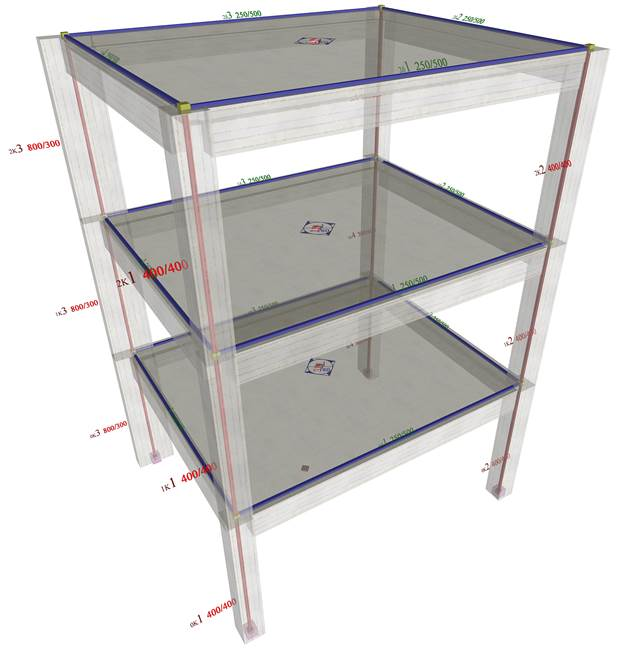# Multistorey-space framesThe structural frame
The construction
The reinforcement I
The reinforcement II
Quantity/Cost estimation
Detailing drawings
Introduction >Wind and Seismic Forces >
Structural model and Analysis
Slabs
Seismic behavour of frames
Appendix A
Appendix B
Appendix C
Appendix D
Introduction >
Modelling slabsMaterials
To be continued >
Introduction

## Multistorey space frame of rectangular columns in parallel arrangementIn this paragraph the analysis of the multistorey space frame illustrated in the figure, under hori-zontal seismic force, is performed using four methods: (i) analysis using manual calculations, assuming fixed-ended columns, (ii) analysis using the Excel file, assuming fixed-ended columns, (iii) analysis using the Excel file, assuming columns with k=6 and (iv) analysis using software, assuming actual beam and column torsional stiffnesses.
This case assuming actual beam and column torsional stiffnesses, cannot be solved by means of manual calculations. The use of software is required and in fact it goes back to the general case which is described in Appendix D, where, in general, the angles a of the principal systems of the storeys are non-zero. In Appendix D a relevant example is also presented for the verifica-tion of the algorithms of the general method.